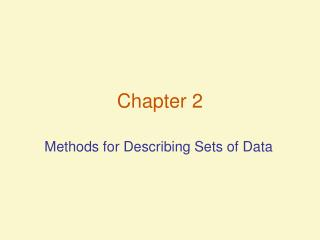DownloadDownload PresentationChapter 2

Chapter 2

Download PresentationChapter 2

- - - - - - - - - - - - - - - - - - - - - - - - - - - E N D - - - - - - - - - - - - - - - - - - - - - - - - - - -
Presentation Transcript

1. Chapter 2 Methods for Describing Sets of Data

2. Objectives • Describe Data using Graphs • Describe Data using Charts

3. Describing Qualitative Data • Qualitative data are nonnumeric in nature • Best described by using Classes • 2 descriptive measures • class frequency – number of data points in a class • class relative = class frequency • frequency total number of data points in data set • class percentage – class relative freq. x 100

4. Describing Qualitative Data – Displaying Descriptive Measures • Summary Table Class Frequency Class percentage – class relative frequency x 100

5. Describing Qualitative Data – Qualitative Data Displays • Pareto Diagram

6. Graphical Methods for Describing Quantitative Data • For describing, summarizing, and detecting patterns in such data, we can use three graphical methods: • dot plots • stem-and-leaf displays • histograms

7. Graphical Methods for Describing Quantitative Data • Stem-and-Leaf Display

8. Graphical Methods for Describing Quantitative Data • More on Histograms

9. Summation Notation • Used to simplify summation instructions • Each observation in a data set is identified by a subscript • x1, x2, x3, x4, x5, …. xn • Notation used to sum the above numbers together is

10. Summation Notation • Data set of 1, 2, 3, 4 • Are these the same? and

11. Numerical Measures of Central Tendency • Central Tendency – tendency of data to center about certain numerical values • 3 commonly used measures of Central Tendency: • Mean • Median • Mode

12. Numerical Measures of Central Tendency • The Mean • Arithmetic average of the elements of the data set • Sample mean denoted by • Population mean denoted by • Calculated as and

13. Numerical Measures of Central Tendency • The Median • Middle number when observations are arranged in order • Median denoted by m • Identified as the observation if n is odd, and the mean of the and observations if n is even

14. Numerical Measures of Central Tendency • The Mode • The most frequently occurring value in the data set • Data set can be multi-modal – have more than one mode • Data displayed in a histogram will have a modal class – the class with the largest frequency

15. Numerical Measures of Central Tendency • The Data set 1 3 5 6 8 8 9 11 12 • Mean • Median is the or 5th observation, 8 • Mode is 8

16. Numerical Measures of Variability • Variability – the spread of the data across possible values • 3 commonly used measures of Variability: • Range • Variance • Standard Deviation

17. Numerical Measures of Variability • The Range • Largest measurement minus the smallest measurement • Loses sensitivity when data sets are large • These 2 distributionshave the same range. • How much does therange tell you about the data variability?

18. Numerical Measures of Variability • The Sample Variance (s2) • The sum of the squared deviations from the mean divided by (n-1). Expressed as units squared • Why square the deviations? The sum of the deviations from the mean is zero

19. Numerical Measures of Variability • The Sample Standard Deviation (s) • The positive square root of the sample variance • Expressed in the original units of measurement

20. Numerical Measures of Variability • Samples and Populations - Notation

21. Numerical Measures of Relative Standing • Descriptive measures of relationship of a measurement to the rest of the data • Common measures: • percentile ranking • z-score

22. Numerical Measures of Relative Standing • Percentile rankings make use of the pth percentile • The median is an example of percentiles. • Median is the 50th percentile – 50 % of observations lie above it, and 50% lie below it • For any p, the pth percentile has p% of the measures lying below it, and (100-p)% above it

23. Numerical Measures of Relative Standing • z-score – the distance between a measurement x and the mean, expressed in standard units • Use of standard units allows comparison across data sets

24. Numerical Measures of Relative Standing • More on z-scores • Z-scores follow the empirical rule for mounded distributions

25. Methods for Detecting Outliers • Outlier – an observation that is unusually large or small relative to the data values being described • Causes: • Invalid measurement • Misclassified measurement • A rare (chance) event • 2 detection methods: • Box Plots • z-scores

26. Methods for Detecting Outliers • Box Plots • based on quartiles, values that divide the dataset into 4 groups • Lower Quartile QL – 25th percentile • Middle Quartile - median • Upper Quartile QU – 75th percentile • Interquartile Range (IQR) = QU - QL

27. Potential Outlier QU (hinge) Whiskers Median QL (hinge) Methods for Detecting Outliers • Box Plots • Not on plot – inner and outer fences, which determine potential outliers

28. Methods for Detecting Outliers • Rules of thumb • Box Plots • measurements between inner and outer fences are suspect • measurements beyond outer fences are highly suspect • Z-scores • Scores of 3 in mounded distributions (2 in highly skewed distributions) are considered outliers